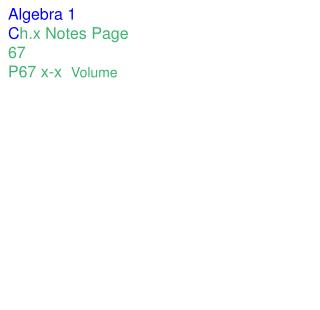DownloadDownload PresentationAlgebra 1 C h.x Notes Page 67 P67 x-x Volume

# Algebra 1 C h.x Notes Page 67 P67 x-x Volume

Download Presentation## Algebra 1 C h.x Notes Page 67 P67 x-x Volume

- - - - - - - - - - - - - - - - - - - - - - - - - - - E N D - - - - - - - - - - - - - - - - - - - - - - - - - - -
##### Presentation Transcript

1. Algebra 1 Ch.x Notes Page 67 P67 x-x Volume

2. Volume is a measurement of... the amount of stuff something can hold. It is measured in Cubic Units. Unit Cube

3. Volume = B x H B depends on the Base Big B = Area of the BASE BASES are Parallel and Congruent (It does not have to be what it is sitting on.) Big H = Height of the Figure HEIGHT is the Distance between the Bases

4. Volume = B x H B depends on the Base Big B = Area of the BASE BASES are Parallel and Congruent (It does not have to be what it is sitting on.) Big H = Height of the Figure HEIGHT is the Distance between the Bases

5. Examples

6. Volume of a Sphere 4πr3 3